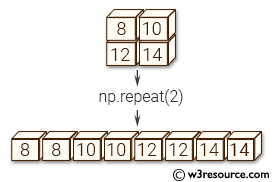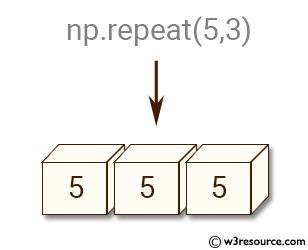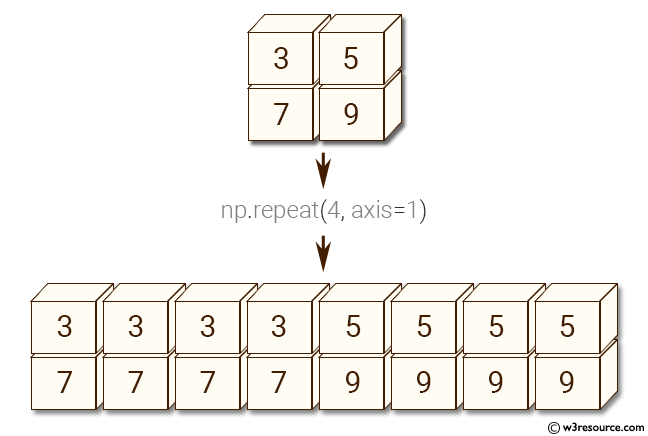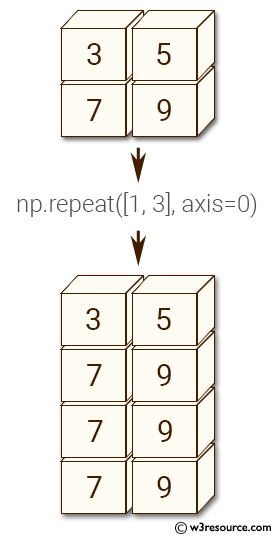# NumPy Array manipulation: repeat() function

## numpy.repeat() function

The repeat() function is used to repeat elements of an array.

Syntax:

`numpy.repeat(a, repeats, axis=None)`Version: 1.15.0

Parameter:

Name Description Required /
Optional
a Input array. Required
repeats The number of repetitions for each element. repeats is broadcasted to fit the shape of the given axis. Required
axis The axis along which to repeat values. By default, use the flattened input array, and return a flat output array. Optional

Return value:

repeated_array [ndarray] Output array which has the same shape as a, except along the given axis.

Example-1: numpy.repeat() function

``````>>> import numpy as np
>>> np.repeat(5,3)
array([5, 5, 5])
``````

Pictorial Presentation:Example-2: numpy.repeat() function

``````>>> import numpy as np
>>> a = np.array([[3,5], [7,9]])
>>> np.repeat(a, 4)
array([3, 3, 3, 3, 5, 5, 5, 5, 7, 7, 7, 7, 9, 9, 9, 9])
>>> np.repeat(a, 4, axis=1)
array([[3, 3, 3, 3, 5, 5, 5, 5],
[7, 7, 7, 7, 9, 9, 9, 9]])
``````

Pictorial Presentation:Example-3: numpy.repeat() function

``````>>> import numpy as np
>>> np.repeat(a, [1,3], axis=0)
array([[3, 5],
[7, 9],
[7, 9],
[7, 9]])
``````

Pictorial Presentation:Python - NumPy Code Editor:

﻿